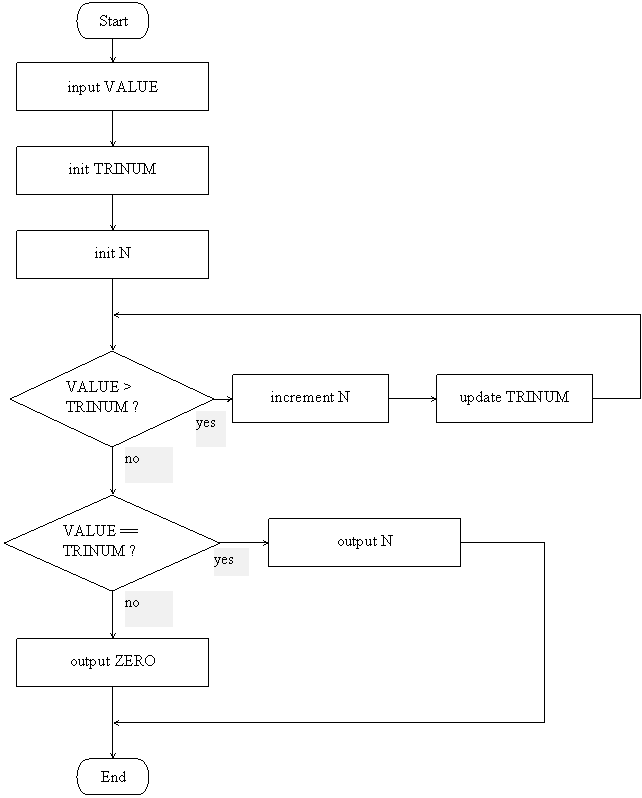# LMC Sample Program

The triangular numbers are as follows:

1     =    1
3     =    1 + 2
6     =    1 + 2 + 3
10    =    1 + 2 + 3 + 4
15    =    1 + 2 + 3 + 4 + 5
21    =    1 + 2 + 3 + 4 + 5 + 6
etc.

The series begins with 1 (the first triangular number).  To calculate the nth triangular number, n is added to the previous triangular number.  For example, the fourth triangular number is calculated by adding 4 to the third triangular number (which is 6), i.e. 10 = (1 + 2 + 3) + 4.

Write a program that takes a single value (greater or equal to zero) as input and outputs which triangular number it is or 0 if it is not a trinangular number.  For example, if the input is 15, the output will be 5 (15 is the 5th triangular number); and if the input is 7, the output will be 0 (7 is not a triangular number).

#### Flowchart

The flowchart for the above program is shown below.#### Program

INP
STA VALUE
LDA ZERO
STA TRINUM
STA N
LOOP LDA TRINUM
SUB VALUE
BRP ENDLOOP
LDA N
STA N
STA TRINUM
BRA LOOP
ENDLOOP LDA VALUE
SUB TRINUM
BRZ EQUAL
LDA ZERO
OUT
BRA DONE
EQUAL LDA N
OUT
DONE HLT
VALUE DAT 000
TRINUM DAT 000
N DAT 000
ZERO DAT 000
ONE DAT 001

#### What you should do

1. Click on the "LMC Simulator Applet" link to start the LMC simulator.
2. Clear the Message Box and all of the LMC mailboxes -- click the "Clear Messages" button and the "Clear" button if necessary.
3. Copy the twenty eight line program above and paste it into the Message Box
4. Click on the "Compile Program" button.
5. Click on the "Run" button.
6. When prompted, enter three-digit numbers in the "In-Box", and press the "Enter" button.

#### What you should see

• After the program is compiled, you should see from mailbox 0 to 23 the instructions 901, 323, 526, 324, 325, 524, 223, 814, 525, 127, 325, 124, 324, 605, 523, 224, 720, 526, 902, 622, 525, 902.  The Program Counter should start at 0 (click on "Reset" if necessary).
• DAT reserves mailbox 23 for N (the user input), mailbox 24 for TRINUM (the value of the current triangular number), mailbox 25 for COUNT (the number of triangular numbers that have been calculated), mailbox 26 for ZERO, and mailbox 27 for ONE.
• When you click on "Run" or "Step", the Message Box will describe the actions of each instruction.
• The first two instructions accept the input VALUE from the user.
• The next three instructions initialize the values of TRINUM and N to ZERO.
• The algorithm is as follows: calculate triangular numbers until the calculated triangular number is greater than or equal to the input value.  If the triangular number is equal to the input, then output N.  Otherwise, output ZERO.
• The LOOP starts by checking this exit condition.  The input VALUE is subtracted from TRINUM.  As long as the result is negative, the LMC stays in the loop (i.e. the BRP does nothing) to calculate more triangular numbers.
• To calculate the next triangular number, N is incremented by ONE, and this new value of N is added to TRINUM.  Remember, the nth triangular number is calculated by adding n to the previous triangular number.
• The BRA LOOP indicates the end of the loop body.  This BRANCH command causes the execution of the LMC to "jump" back to the start of the loop.
• After exiting the LOOP, the value of TRINUM is greater than or equal to the input VALUE -- the LMC has calculated enough triangular numbers to determine if the user input is one.
• The SUB instruction is to check if TRINUM and VALUE are equal.
• If they are equal, subtracting TRINUM from VALUE will equal 000.  BRZ will allow the LMC to skip past the code section that handles when these two values are not equal.  The LMC will then execute the code segment that outputs N to the Out-Box -- the input VALUE is the Nth triangular number.
• If they are not equal, the result on the Accumulator will not be 000, and BRZ will do nothing.  The code after of the BRZ instruction is executed.  This code outputs ZERO to the Out-Box, and then skips past the code for the two values being EQUAL to the end of the program.